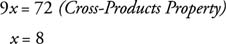## Similar Polygons

Two polygons with the same shape are called similar polygons. The symbol for “is similar to” is ∼. Notice that it is a portion of the “is congruent to” symbol, ≅. When two polygons are similar, these two facts both must be true:

• Corresponding angles are equal.
• The ratios of pairs of corresponding sides must all be equal.This means: m ∠ A = m ∠ Em ∠ B = m ∠ Fm ∠ C = m ∠ Gm ∠ D = m ∠ H, andIt is possible for a polygon to have one of the above facts true without having the other fact true. The following two examples show how that is possible.Figure 2 Quadrilaterals that are not similar to one another.

Even though the ratios of corresponding sides are equal, corresponding angles are not equal (90° ≠ 120°, 90° ≠ 60°).Figure 3 Quadrilaterals that are not similar to one another.

Even though corresponding angles are equal, the ratios of each pair of corresponding sides are not equal (3/3≠5/3).

Example 1: In Figure 4, quadrilateral ABCD ∼ quadrilateral EFGH. (a) Find m ∠ E. (b) Find x.